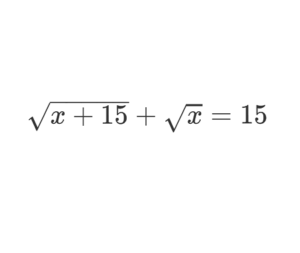Home -> Solved problems -> Solve the equation

## Solve the equation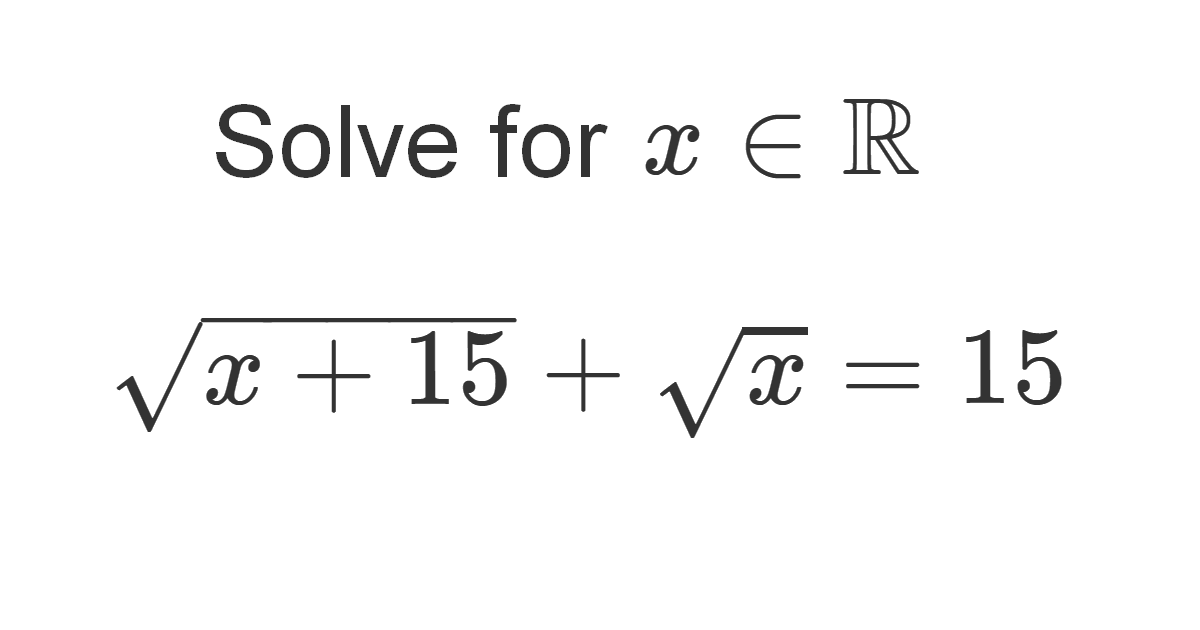### Solution

This is how to solve this type of equation:
\begin{aligned} &\sqrt{x+15}+\sqrt{x}=15 \;\;\;\;\;\;\;\text { } (1) \\\\ &(\sqrt{x+15}+\sqrt{x})(\sqrt{x+15}-\sqrt{x})=15(\sqrt{x+15}-\sqrt{x}) \\\\ &x+15-x=15(\sqrt{x+15}-\sqrt{x}) \\\\ &15=15(\sqrt{x+15}-\sqrt{x}) \\\\ &(\sqrt{x+15}-\sqrt{x})=1 \;\;\;\;\;\;\;\text { } (2)\\\\ &\text { Using } (1) \text { and } (2) \\\\ &2 \sqrt{x+15}=16 \\\\ &\sqrt{x+15}=8 \\\\ &x+15=64 \\\\ &x=49 \end{aligned}
Home -> Solved problems -> Solve the equation

### Related Topics

Find the volume of the square pyramid as a function of $$a$$ and $$H$$ by slicing method.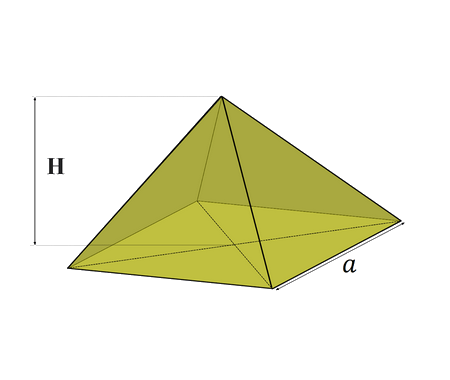Prove that $\lim_{x \rightarrow 0}\frac{\sin x}{x}=1$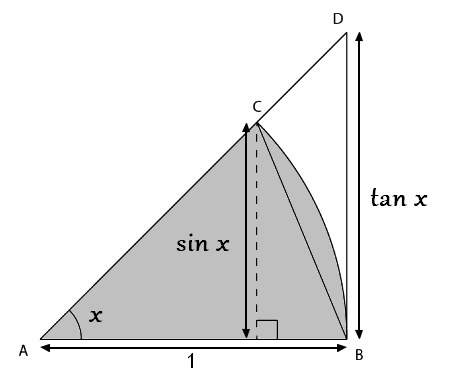Prove that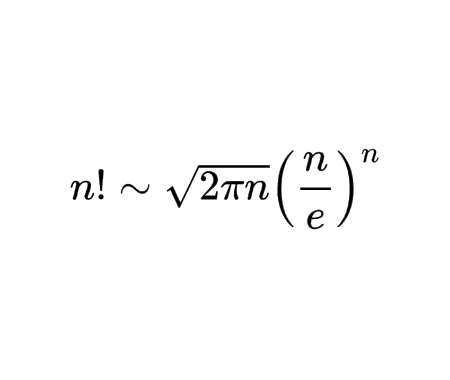Calculate the half derivative of $$x$$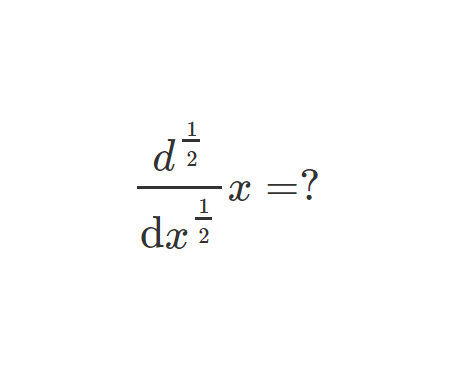Prove Wallis Product Using Integration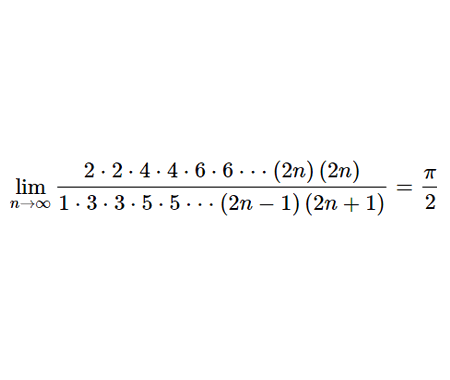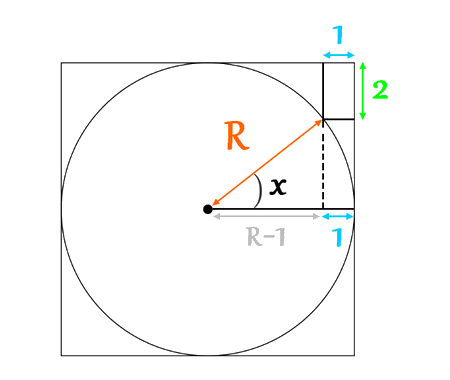Calculate the volume of Torus using cylindrical shells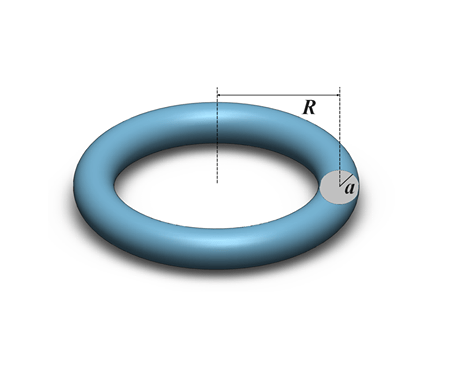Find the derivative of exponential $$x$$ from first principles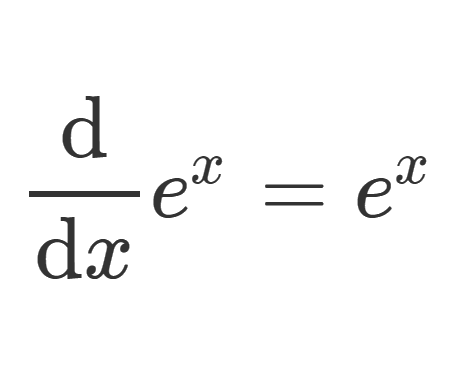Calculate the sum of areas of the three squares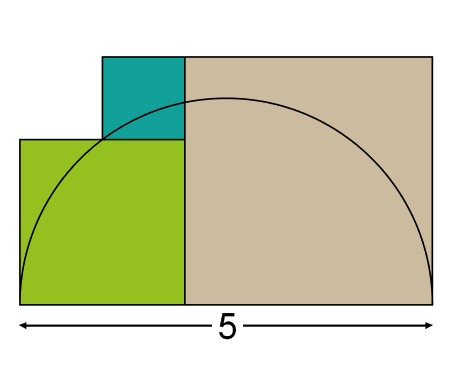Find the equation of the curve formed by a cable suspended between two points at the same height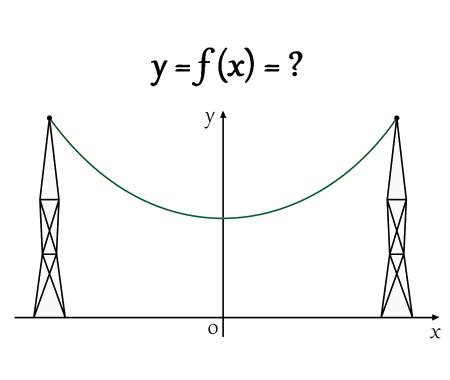Solve the equation for real values of $$x$$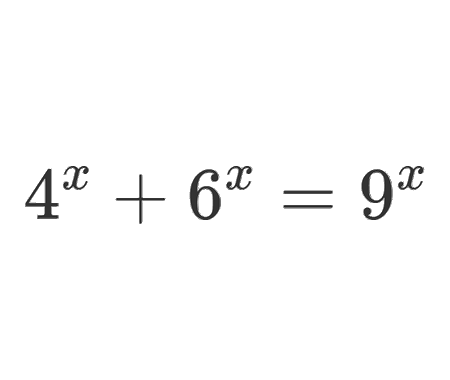Solve the equation for $$x\epsilon\mathbb{R}$$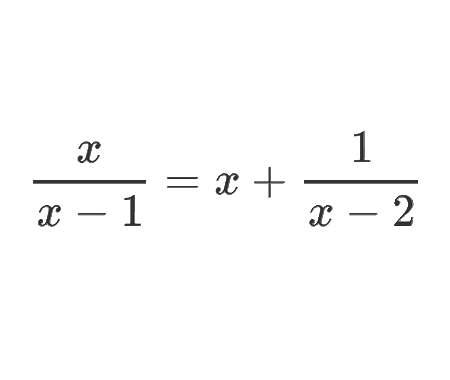Determine the angle $$x$$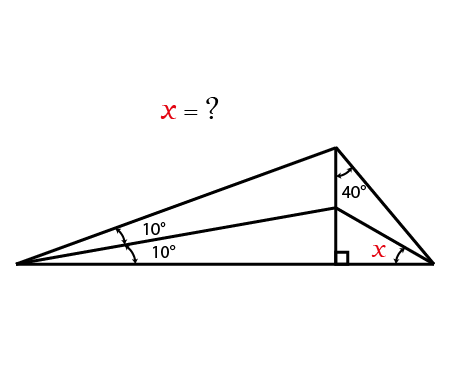Calculate the following limit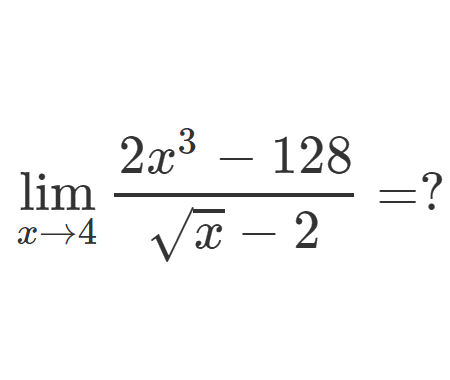Calculate the following limit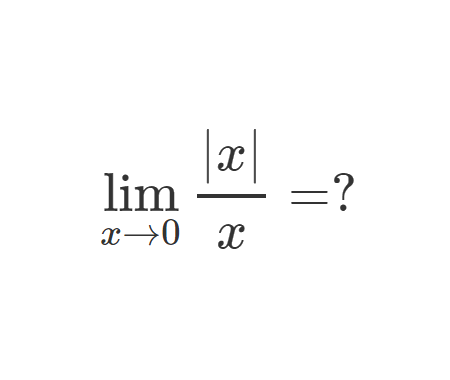Calculate the integral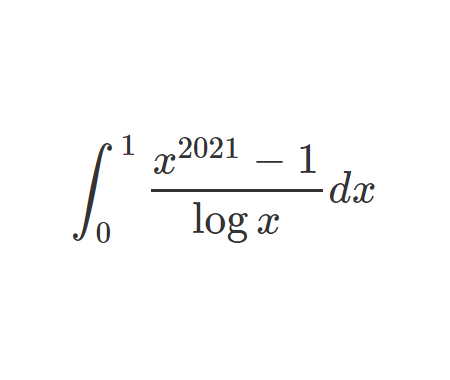Challenging problem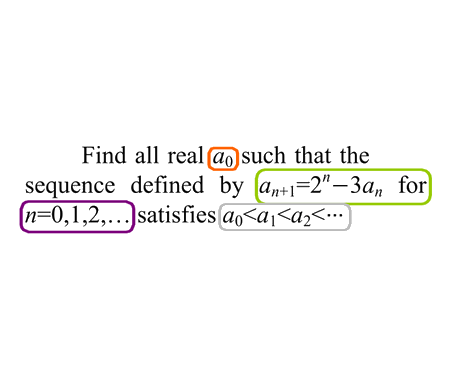Prove that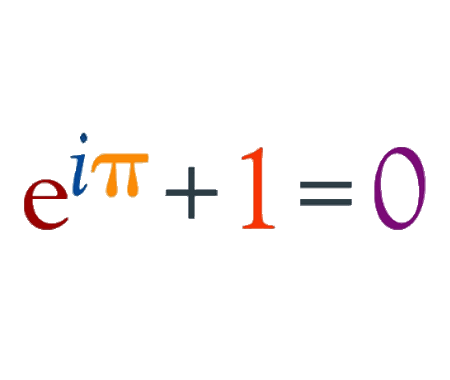Prove that $$e$$ is an irrational number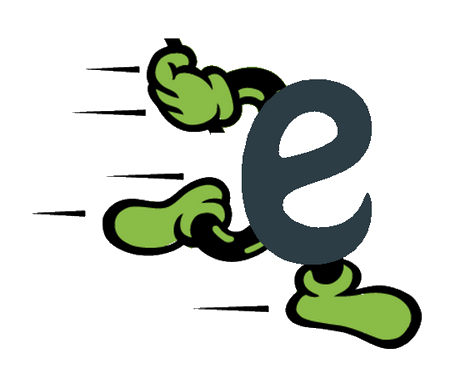Find the derivative of $$y$$ with respect to $$x$$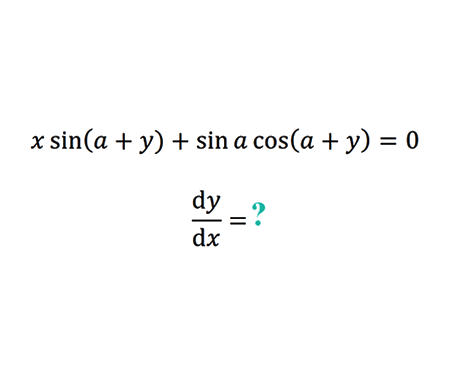Find the limit of width and height ratio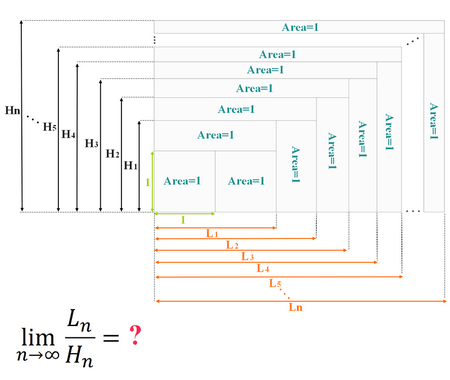How Tall Is The Table ?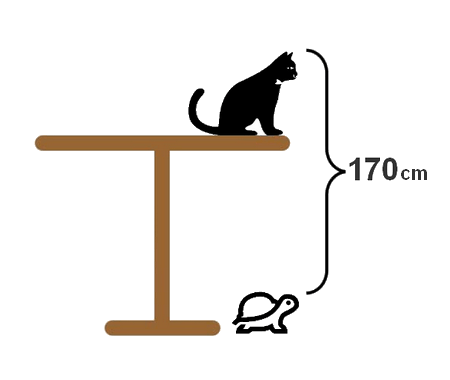Why 0.9999999...=1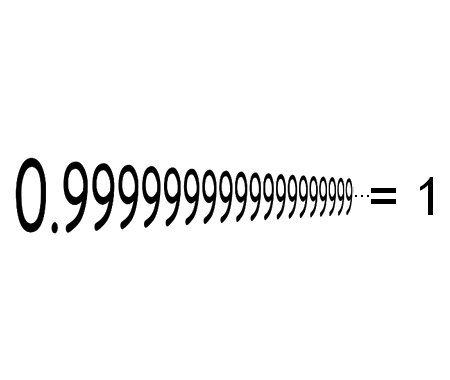Solve the equation for $$x \in \mathbb{R}$$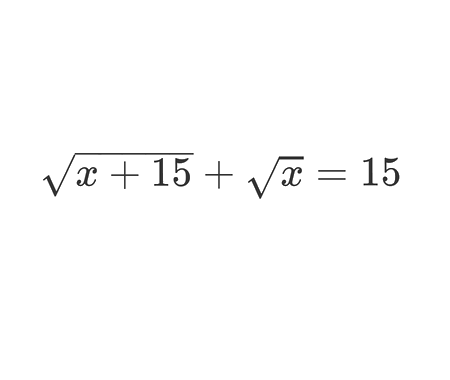Home -> Solved problems -> Solve the equation

#### Share the solution: Solve the equation